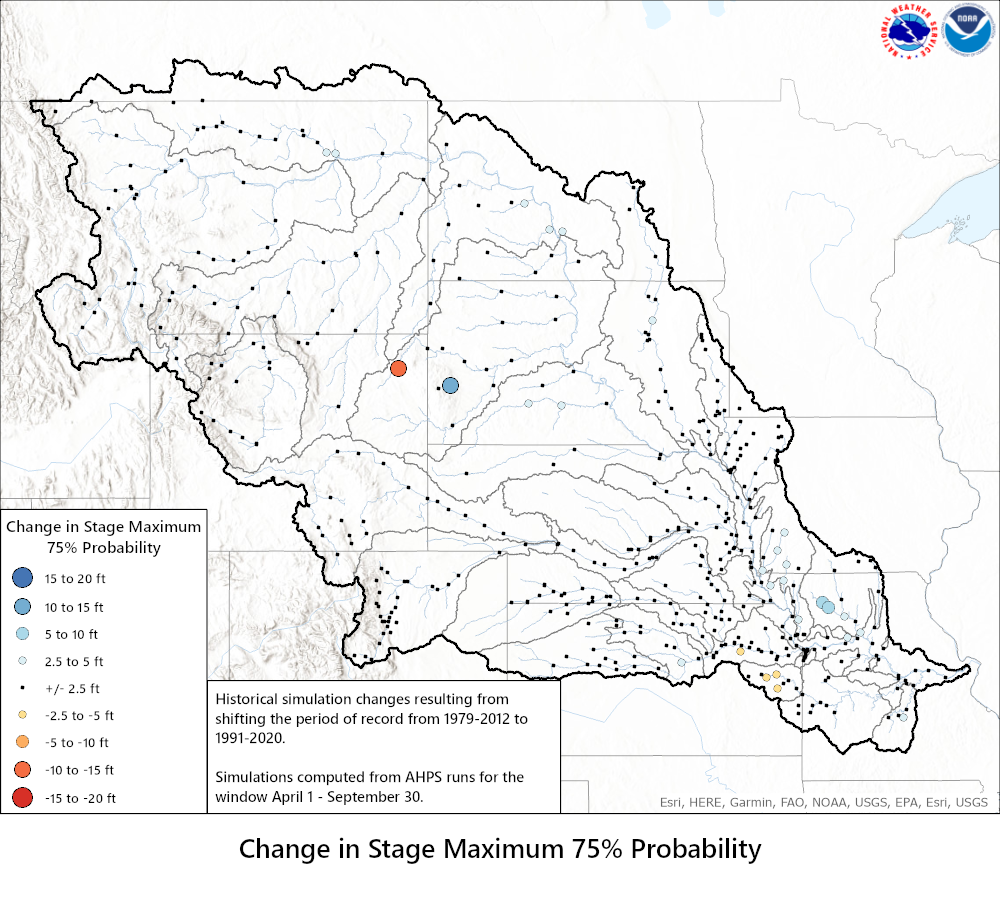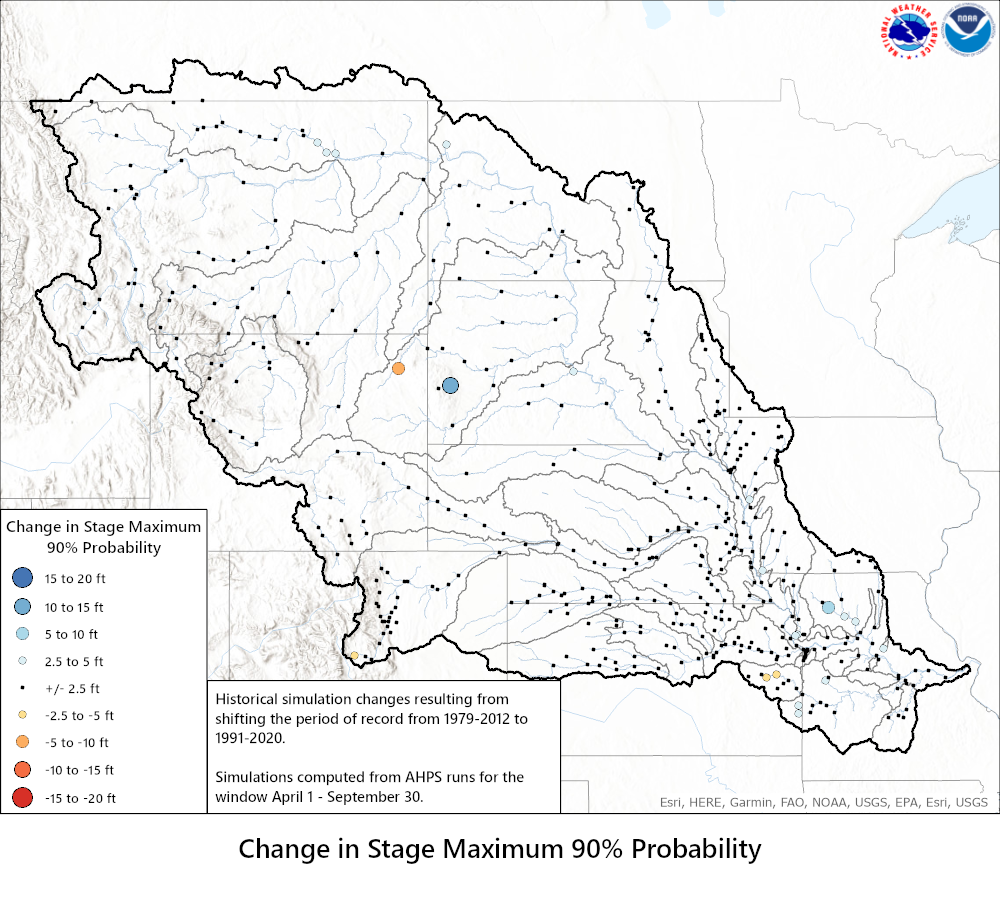## Probability Distributions by Climate Period

 The historical simulation can be defined as the hydrologic model climatology that should reflect the historic range of possibilities. With the historical simulation, the hydrologic modeling system is executed one time for the years of interest. The computation begins using standard starting conditions for snow, soil and runoff conditions. The hydrologic models use only historical temperature and precipitation as the model inputs (forcings).  Using those inputs, the hydrologic models are then executed continuously for the years of interest producing one continuous hydrograph of streamflow for those years. The peak flows for each year are selected for the period of interest (for example March 1 - May 30) and ranked to determine probabilities of exceedance illustrated on this webpage. Probabilities of exceedance can also be produced for other parameters such as volume or stage.   In this study the hydrologic models were executed using two different periods of record to compute the continuous hydrograph described above. The years 1979 through 2012 were the previous years that were used for the temperature and precipitation model inputs to produce the conditional simulations. The conditional simulations are used for the official AHPS probabilistic outlooks. For more information about conditional simulations see, https://www.weather.gov/media/tfx/AHPS_Brochure_Final_Inside_2.pdf and https://water.weather.gov/ahps2/pdf/About_Chance_of_Exceeding_River_Stage_Graphic.pdf. The years 1991 through 2020 are the years now used to produce the conditional simulation AHPS probabilistic outlooks. The intent of this study is just to illustrate how the AHPS probabilistic outlooks might have shifted by comparing the two different computed probabilities of exceedance for peak flow, volume and stage.

Select Forecast Group
 Big Blue Bighorn Elkhorn-Platte Grand James Kansas Loup Lower Dakota Tribs Lower Missouri Tribs Lower Republican Lower Smoky Hill Marais Des Cygnes Middle Dakota Tribs Milk Missouri Mainstem North Platte Osage Siouxs South Platte Upper Dakota Tribs Upper Missouri Upper Missouri Tribs Upper Republican Upper Smoky Hill Yellowstone

 Discharge Volume Change 10% Probability 25% Probability 50% Probability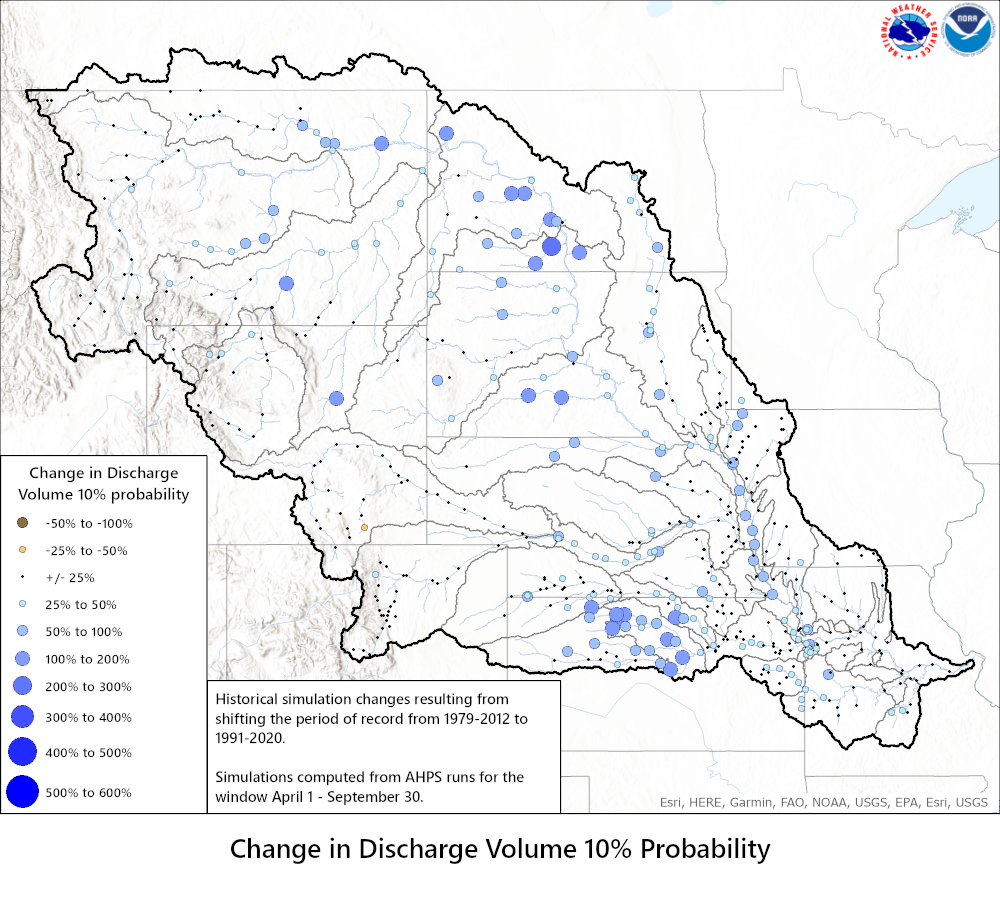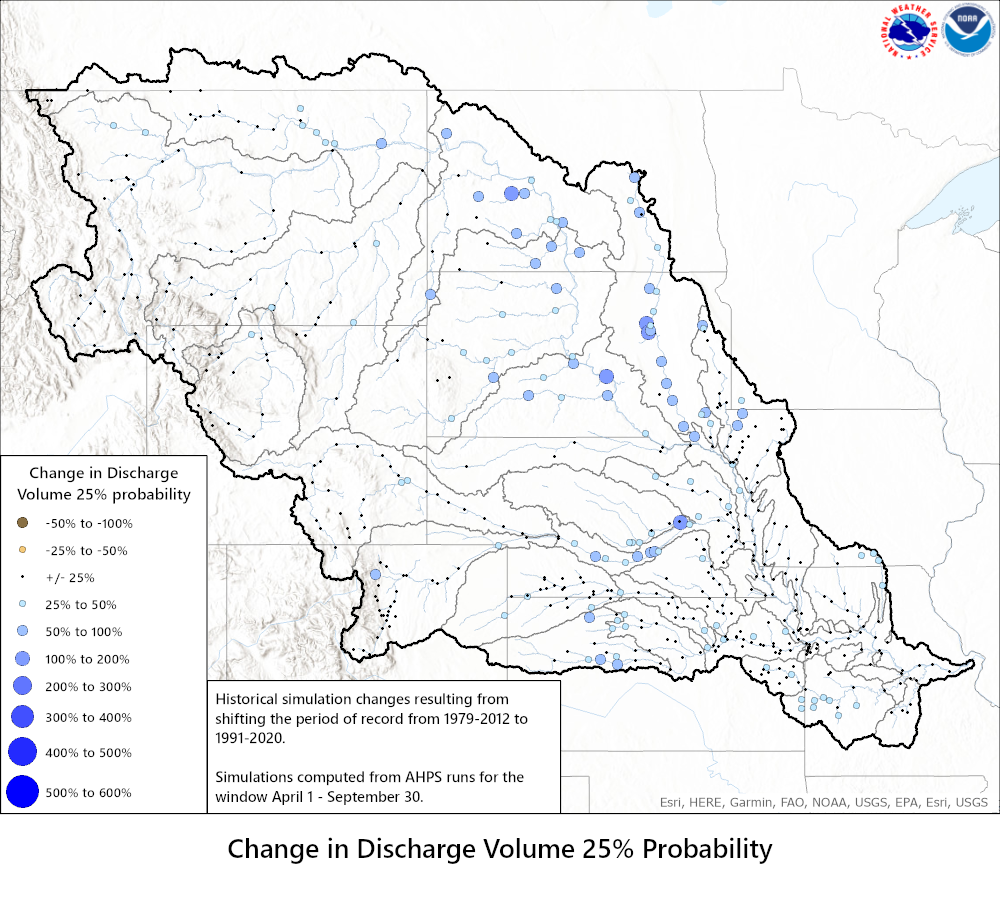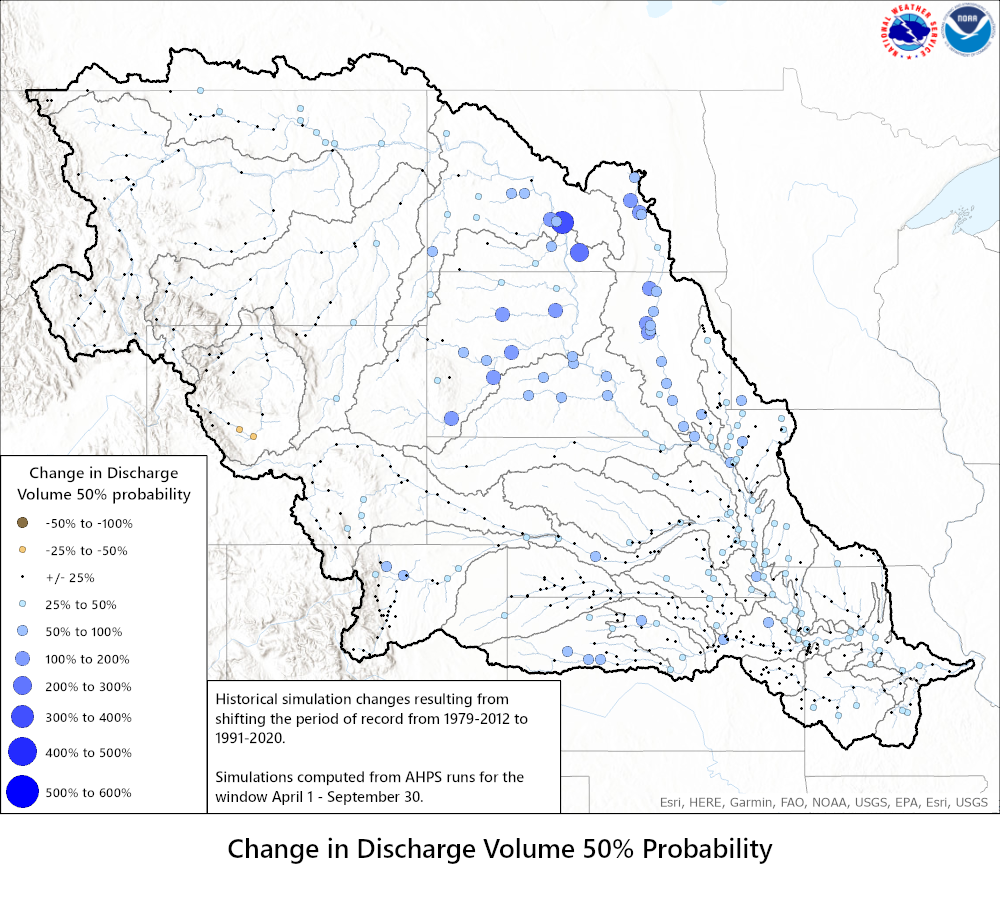75% Probability 90% Probability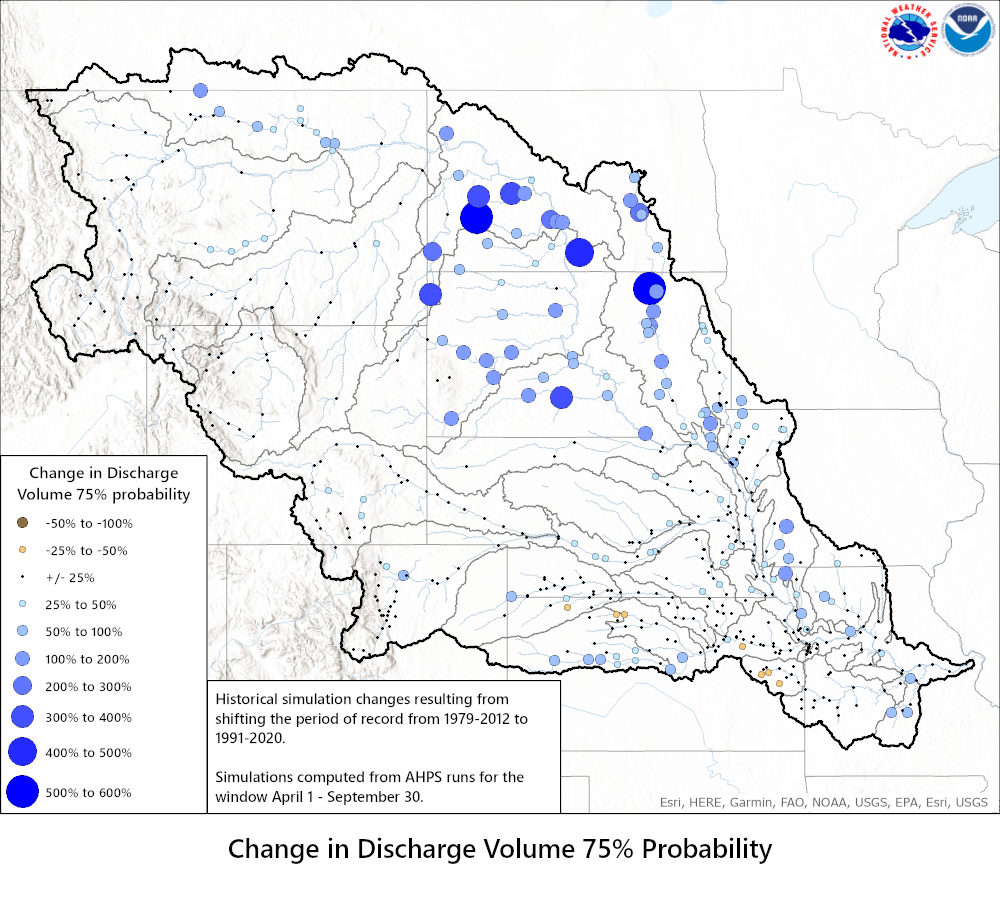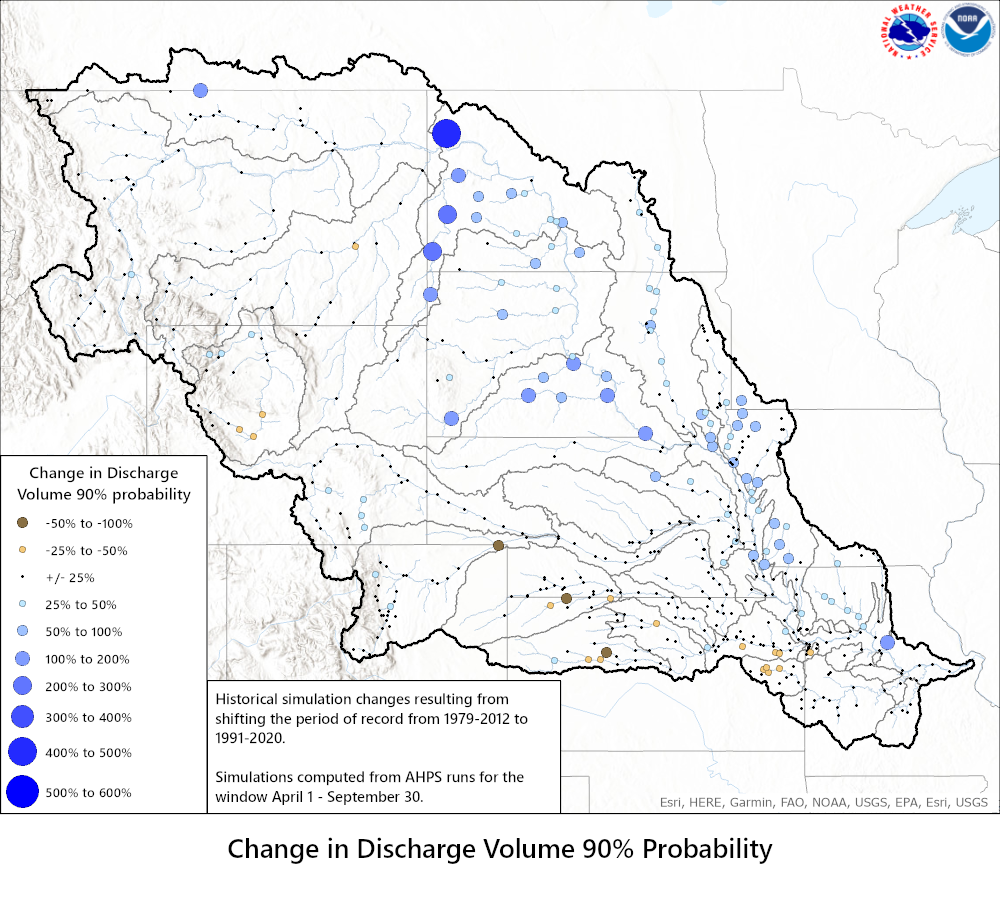Discharge Maximum Change 10% Probability 25% Probability 50% Probability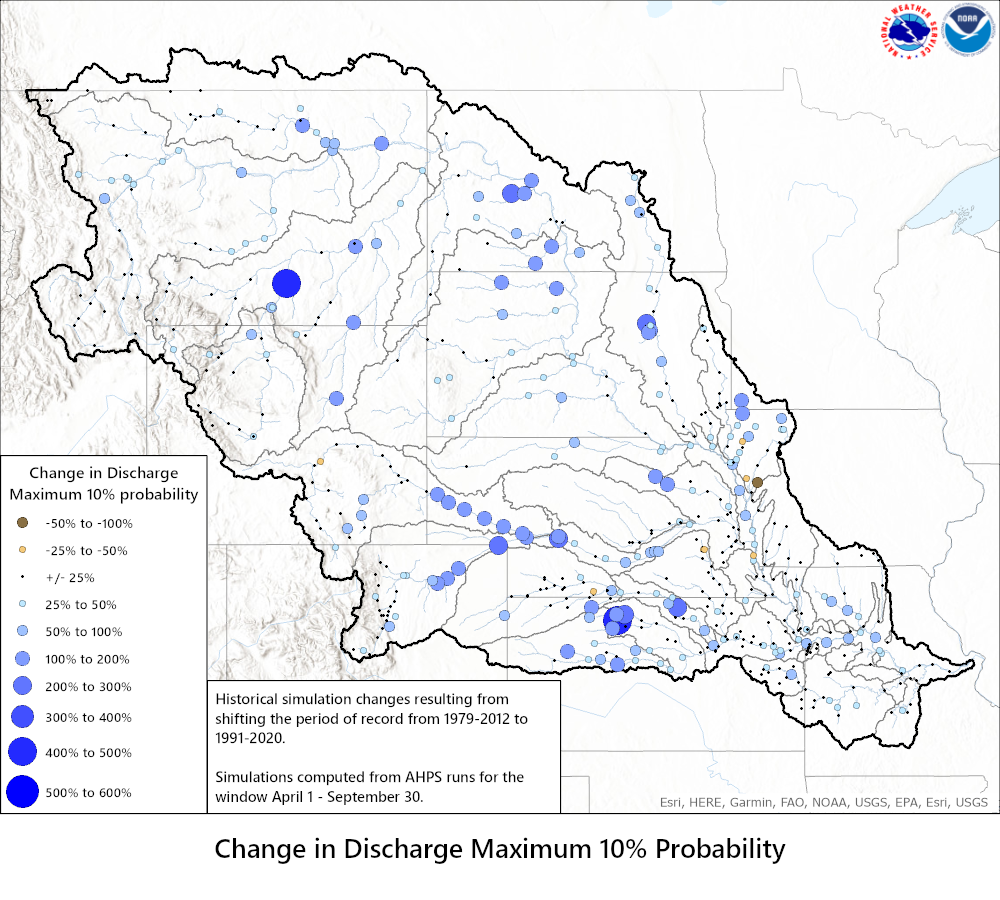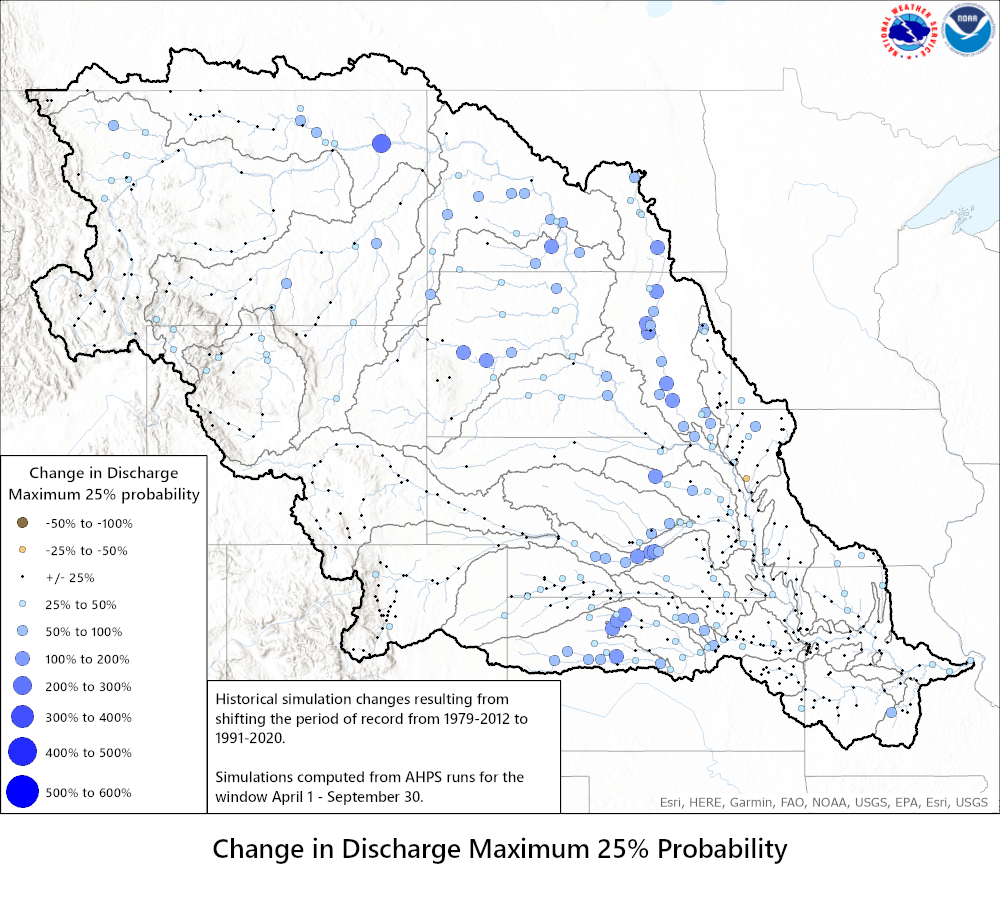75% Probability 90% Probability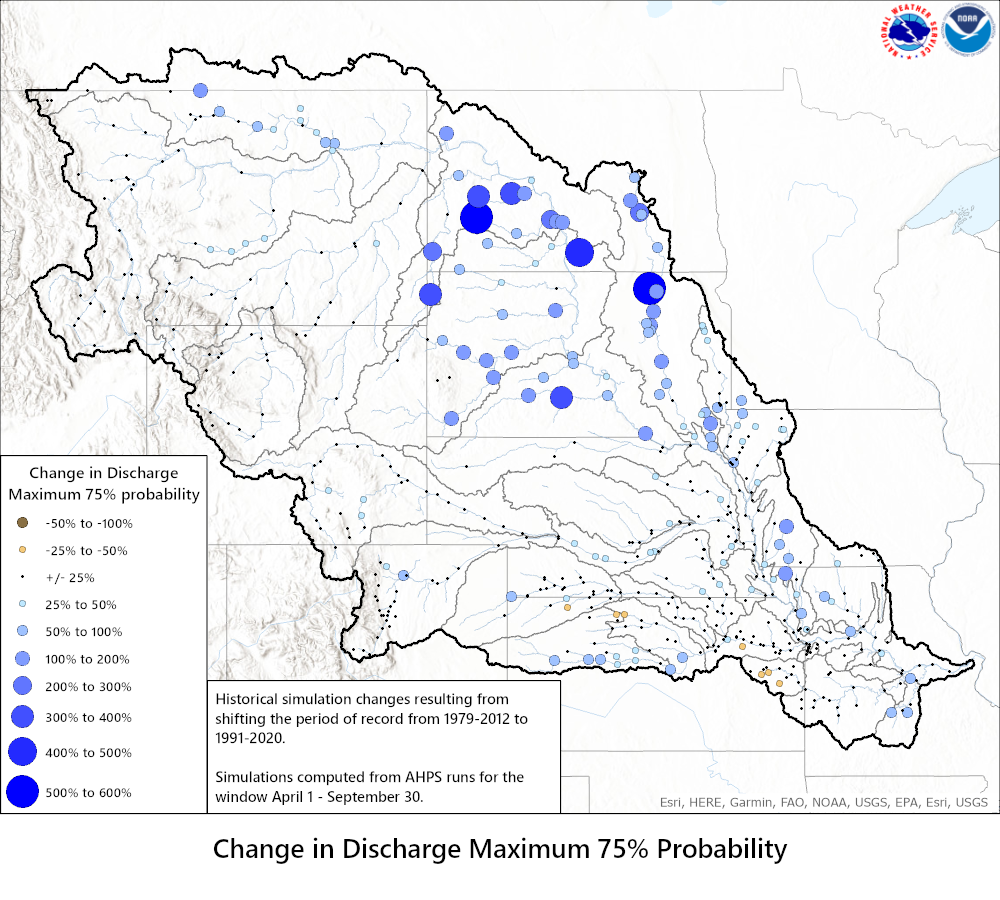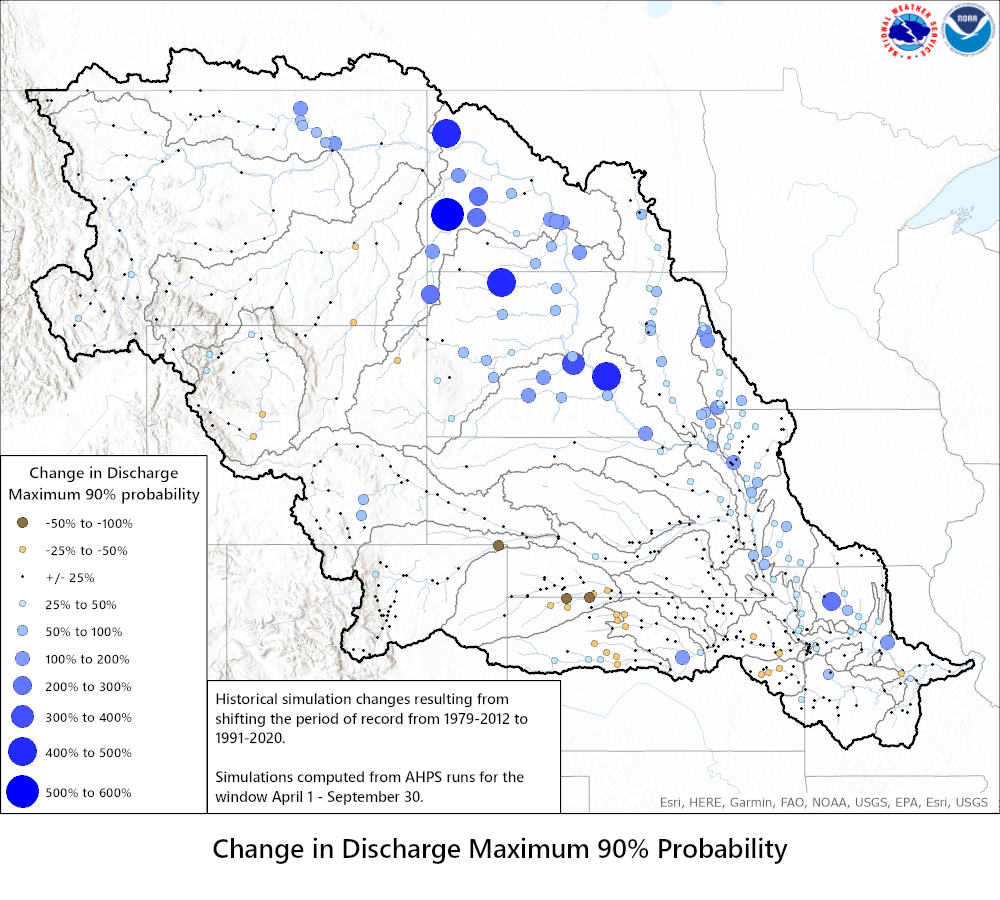Stage Maximum Change 10% Probability 25% Probability 50% Probability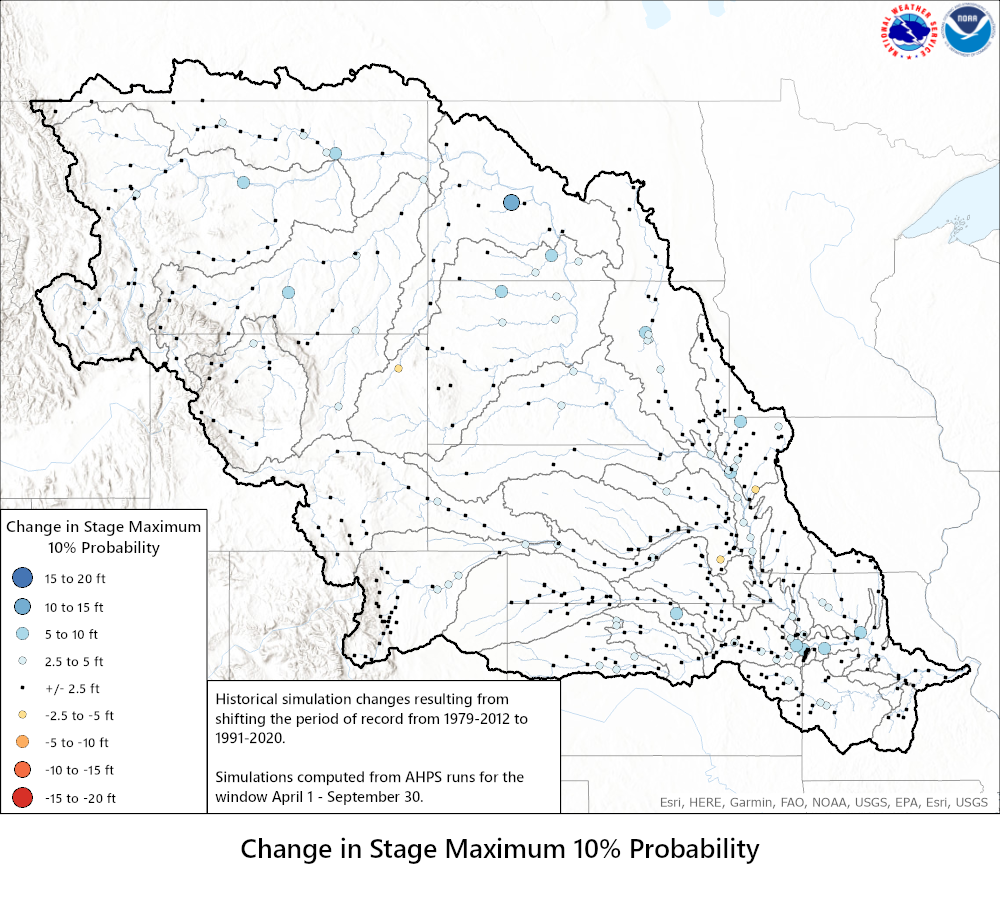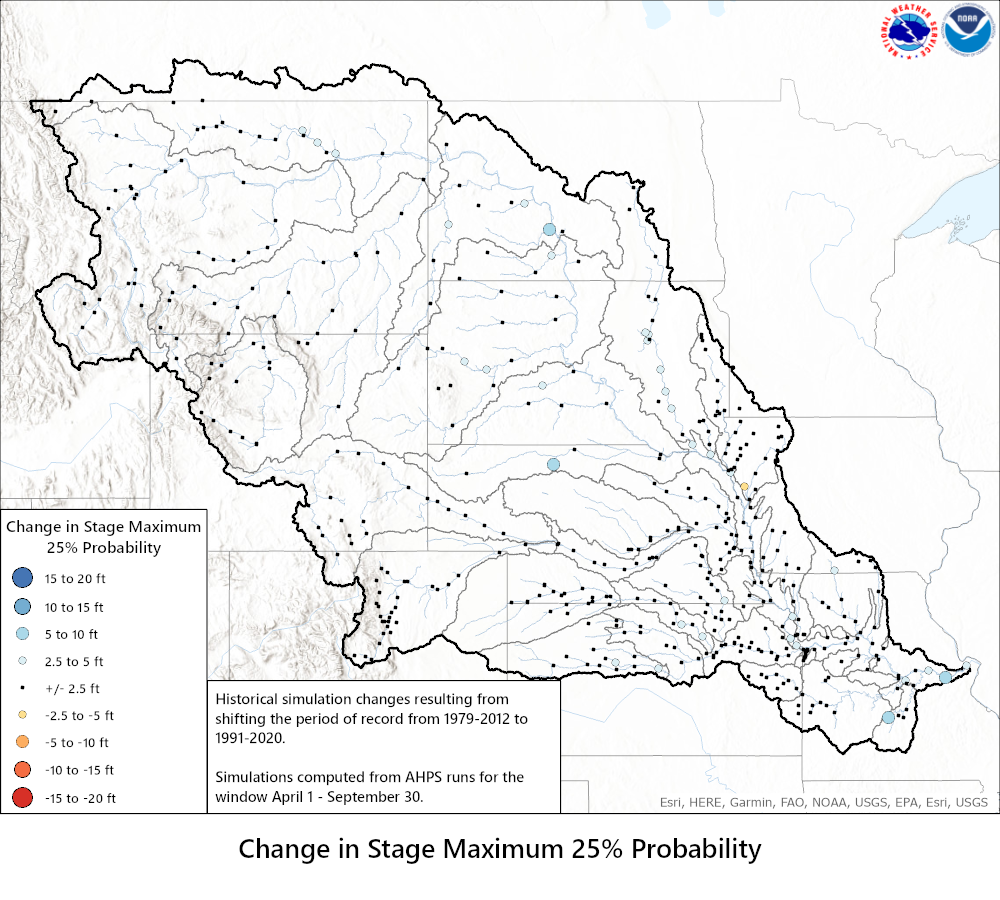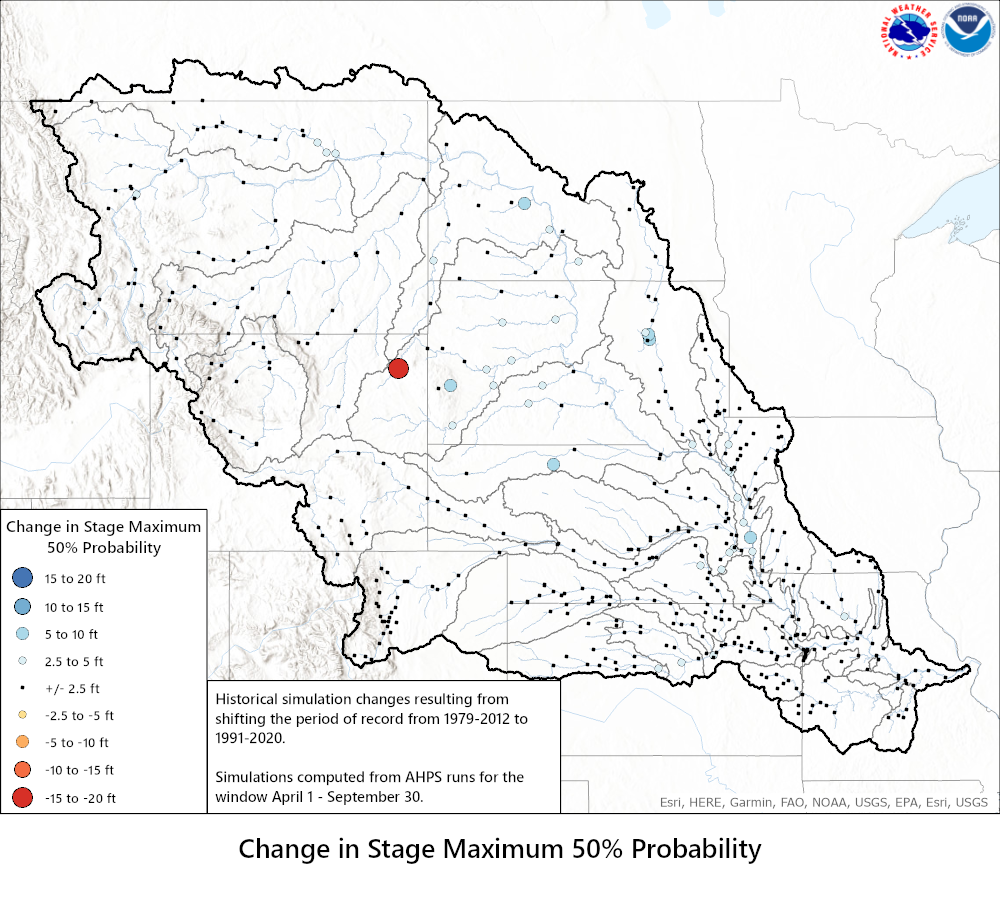75% Probability 90% Probability